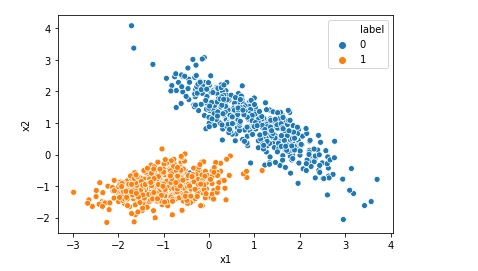# Print ROC AUC Receiver Operating Characteristic Area Under Curve

The receiver operating characteristic area under curve is a way to measure the performance of a classification model, may be created using algorithms like Logistic Regression. ROC-AUC is basically a graph where we plot true positive rate on y-axis and false positive rate on x-axis. If a model is good the AUC will be close to 1. Area … Continue reading Print ROC AUC Receiver Operating Characteristic Area Under Curve# Creating Synthetic Data for Logistic Regression

Many a times we want to implement Logistic Regression on certain data but we do not find that kind of data online. In that case we can generate a synthetic data for our problem. In this post we will see how to generate a typical synthetic data for a simple Logistic Regression. Import the required … Continue reading Creating Synthetic Data for Logistic Regression# Creating Synthetic Data for Linear Regression

Many a times you want to implement Linear Regression on certain data but you do not find that kind of data online. In that case you can generate a synthetic data for your problem. In this post we will see how to generate a typical synthetic data for a simple Linear Regression. Import the required … Continue reading Creating Synthetic Data for Linear Regression# Visualize and Print Confusion Matrix

In many cases you would like to print the confusion matrix in a better format and look and feel than what is provided by scikit learn by default. The default look when printing confusion matrix using scikit learn scikit-learn default confusion matrix print However in many cases you may like to print the confusion matrix … Continue reading Visualize and Print Confusion Matrix# What is Root Mean Squared Error or RMSE

Root mean squared error or RMSE is a measure of the difference between actual values and predicted values of a machine learning model  like Linear Regression. Root mean squared error is a measure of how well the machine learning model can perform. The lower the RMSE, the better the model. RMSE is always positive, and … Continue reading What is Root Mean Squared Error or RMSE# What is R Squared for Linear Regression

For Linear Regression, R-squared is a statistical term which indicates how close the data are to the fitted regression line. R-Squared is also known as coefficient of determination. R-squared = Explained variation in data / Total variation in data R-squared = 1 - (RSS/TSS) RSS = Sum of squares of difference between predicted value and … Continue reading What is R Squared for Linear Regression# What is Precision, Recall and F1 Score

Precision, recall and F1 Score are parameters to measure the performance of a classification model. For example if we want to implement Logistic Regression model on an imbalanced dataset we would like to calculate precision, recall and F1 score as accuracy may not be a good measure of model performance in this case. Precision: Precision … Continue reading What is Precision, Recall and F1 Score# What is Confusion Matrix in Machine Learning

Not only human beings but also the machine learning models may get confused !! After all Artificial Intelligence mimics a human brain, isn’t it?(pun intended). Imagine yourself as a machine learning engineer and suppose you trained a machine learning classification model successfully today. After the model is trained you checked the accuracy which is 93.0%. Wow … Continue reading What is Confusion Matrix in Machine Learning# Difference between Logistic Regression and Support Vector Machine SVM

Both Logistic Regression and Support Vector Machine or SVM can be used for classification however there is some fundamental difference between them. Lets understand those differences when we are using Support Vector Machine as a classifier. Logistic Regression gives probabilities as output that can be interpreted as confidence in the classification decision however Support Vector … Continue reading Difference between Logistic Regression and Support Vector Machine SVM# Top 5 Assumptions of Linear Regression Algorithm

We can not implement Linear Regression on any given data. If we have got a dataset and we are planning to implement Linear Regression Model on that data we must first check if the given data is inline with the assumptions of Linear Regression. Once the given data agrees to all the assumptions then only … Continue reading Top 5 Assumptions of Linear Regression Algorithm